# Value-at-Risk Estimation and Backtesting

This example shows how to estimate the value-at-risk (VaR) using three methods and perform a VaR backtesting analysis. The three methods are:

1. Normal distribution

2. Historical simulation

3. Exponential weighted moving average (EWMA)

Value-at-risk is a statistical method that quantifies the risk level associated with a portfolio. The VaR measures the maximum amount of loss over a specified time horizon and at a given confidence level.

Backtesting measures the accuracy of the VaR calculations. Using VaR methods, the loss forecast is calculated and then compared to the actual losses at the end of the next day. The degree of difference between the predicted and actual losses indicates whether the VaR model is underestimating or overestimating the risk. As such, backtesting looks retrospectively at data and helps to assess the VaR model.

The three estimation methods used in this example estimate the VaR at 95% and 99% confidence levels.

### Load the Data and Define the Test Window

Load the data. The data used in this example is from a time series of returns on the S&P index from 1993 through 2003.

load VaRExampleData.mat Returns = tick2ret(sp); DateReturns = dates(2:end); SampleSize = length(Returns); 

Define the estimation window as 250 trading days. The test window starts on the first day in 1996 and runs through the end of the sample.

TestWindowStart = find(year(DateReturns)==1996,1); TestWindow = TestWindowStart : SampleSize; EstimationWindowSize = 250; 

For a VaR confidence level of 95% and 99%, set the complement of the VaR level.

pVaR = [0.05 0.01]; 

These values mean that there is at most a 5% and 1% probability, respectively, that the loss incurred will be greater than the maximum threshold (that is, greater than the VaR).

### Compute the VaR Using the Normal Distribution Method

For the normal distribution method, assume that the profit and loss of the portfolio is normally distributed. Using this assumption, compute the VaR by multiplying the z-score, at each confidence level by the standard deviation of the returns. Because VaR backtesting looks retrospectively at data, the VaR "today" is computed based on values of the returns in the last N = 250 days leading to, but not including, "today."

Zscore = norminv(pVaR); Normal95 = zeros(length(TestWindow),1); Normal99 = zeros(length(TestWindow),1); for t = TestWindow i = t - TestWindowStart + 1; EstimationWindow = t-EstimationWindowSize:t-1; Sigma = std(Returns(EstimationWindow)); Normal95(i) = -Zscore(1)*Sigma; Normal99(i) = -Zscore(2)*Sigma; end figure; plot(DateReturns(TestWindow),[Normal95 Normal99]) xlabel('Date') ylabel('VaR') legend({'95% Confidence Level','99% Confidence Level'},'Location','Best') title('VaR Estimation Using the Normal Distribution Method')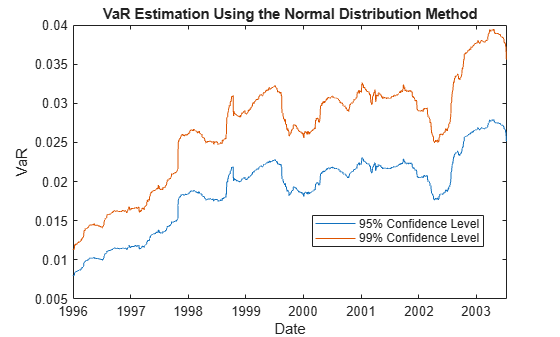The normal distribution method is also known as parametric VaR because its estimation involves computing a parameter for the standard deviation of the returns. The advantage of the normal distribution method is its simplicity. However, the weakness of the normal distribution method is the assumption that returns are normally distributed. Another name for the normal distribution method is the variance-covariance approach.

### Compute the VaR Using the Historical Simulation Method

Unlike the normal distribution method, the historical simulation (HS) is a nonparametric method. It does not assume a particular distribution of the asset returns. Historical simulation forecasts risk by assuming that past profits and losses can be used as the distribution of profits and losses for the next period of returns. The VaR "today" is computed as the p th-quantile of the last N returns prior to "today."

Historical95 = zeros(length(TestWindow),1); Historical99 = zeros(length(TestWindow),1); for t = TestWindow i = t - TestWindowStart + 1; EstimationWindow = t-EstimationWindowSize:t-1; X = Returns(EstimationWindow); Historical95(i) = -quantile(X,pVaR(1)); Historical99(i) = -quantile(X,pVaR(2)); end figure; plot(DateReturns(TestWindow),[Historical95 Historical99]) ylabel('VaR') xlabel('Date') legend({'95% Confidence Level','99% Confidence Level'},'Location','Best') title('VaR Estimation Using the Historical Simulation Method')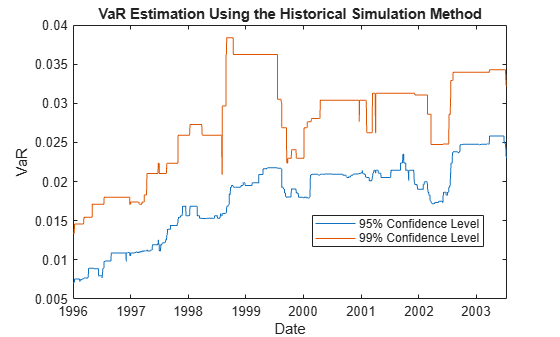The preceding figure shows that the historical simulation curve has a piecewise constant profile. The reason for this is that quantiles do not change for several days until extreme events occur. Thus, the historical simulation method is slow to react to changes in volatility.

### Compute the VaR Using the Exponential Weighted Moving Average Method (EWMA)

The first two VaR methods assume that all past returns carry the same weight. The exponential weighted moving average (EWMA) method assigns nonequal weights, particularly exponentially decreasing weights. The most recent returns have higher weights because they influence "today's" return more heavily than returns further in the past. The formula for the EWMA variance over an estimation window of sizeis:whereis a normalizing constant:For convenience, we assume an infinitely large estimation window to approximate the variance:A value of the decay factor frequently used in practice is 0.94. This is the value used in this example. For more information, see References.

Initiate the EWMA using a warm-up phase to set up the standard deviation.

Lambda = 0.94; Sigma2 = zeros(length(Returns),1); Sigma2(1) = Returns(1)^2; for i = 2 : (TestWindowStart-1) Sigma2(i) = (1-Lambda) * Returns(i-1)^2 + Lambda * Sigma2(i-1); end 

Use the EWMA in the test window to estimate the VaR.

Zscore = norminv(pVaR); EWMA95 = zeros(length(TestWindow),1); EWMA99 = zeros(length(TestWindow),1); for t = TestWindow k = t - TestWindowStart + 1; Sigma2(t) = (1-Lambda) * Returns(t-1)^2 + Lambda * Sigma2(t-1); Sigma = sqrt(Sigma2(t)); EWMA95(k) = -Zscore(1)*Sigma; EWMA99(k) = -Zscore(2)*Sigma; end figure; plot(DateReturns(TestWindow),[EWMA95 EWMA99]) ylabel('VaR') xlabel('Date') legend({'95% Confidence Level','99% Confidence Level'},'Location','Best') title('VaR Estimation Using the EWMA Method')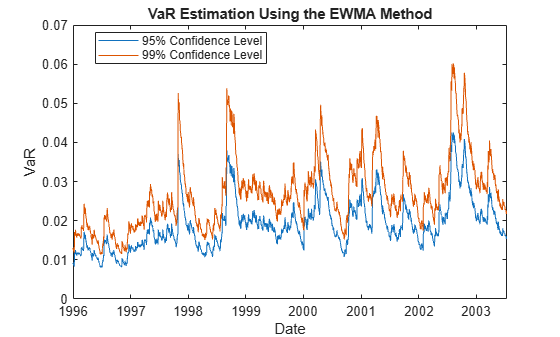In the preceding figure, the EWMA reacts very quickly to periods of large (or small) returns.

### VaR Backtesting

In the first part of this example, VaR was estimated over the test window with three different methods and at two different VaR confidence levels. The goal of VaR backtesting is to evaluate the performance of VaR models. A VaR estimate at 95% confidence is violated only about 5% of the time, and VaR failures do not cluster. Clustering of VaR failures indicates the lack of independence across time because the VaR models are slow to react to changing market conditions.

A common first step in VaR backtesting analysis is to plot the returns and the VaR estimates together. Plot all three methods at the 95% confidence level and compare them to the returns.

ReturnsTest = Returns(TestWindow); DatesTest = DateReturns(TestWindow); figure; plot(DatesTest,[ReturnsTest -Normal95 -Historical95 -EWMA95]) ylabel('VaR') xlabel('Date') legend({'Returns','Normal','Historical','EWMA'},'Location','Best') title('Comparison of returns and VaR at 95% for different models')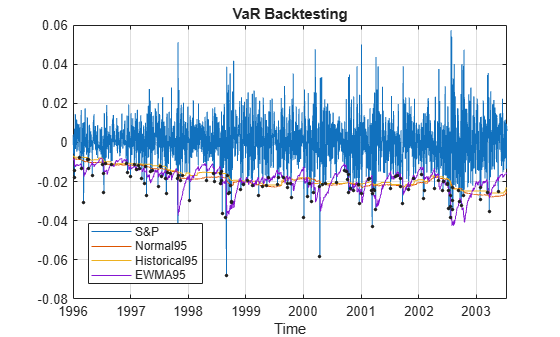To highlight how the different approaches react differently to changing market conditions, you can zoom in on the time series where there is a large and sudden change in the value of returns. For example, around August 1998:

ZoomInd = (DatesTest >= datestr('5-Aug-1998','local')) & (DatesTest <= datestr('31-Oct-1998','local')); VaRData = [-Normal95(ZoomInd) -Historical95(ZoomInd) -EWMA95(ZoomInd)]; VaRFormat = {'-','--','-.'}; D = DatesTest(ZoomInd); R = ReturnsTest(ZoomInd); N = Normal95(ZoomInd); H = Historical95(ZoomInd); E = EWMA95(ZoomInd); IndN95 = (R < -N); IndHS95 = (R < -H); IndEWMA95 = (R < -E); figure; bar(D,R,0.5,'FaceColor',[0.7 0.7 0.7]); hold on for i = 1 : size(VaRData,2) stairs(D-0.5,VaRData(:,i),VaRFormat{i}); end ylabel('VaR') xlabel('Date') legend({'Returns','Normal','Historical','EWMA'},'Location','Best','AutoUpdate','Off') title('95% VaR violations for different models') ax = gca; ax.ColorOrderIndex = 1; plot(D(IndN95),-N(IndN95),'o',D(IndHS95),-H(IndHS95),'o',... D(IndEWMA95),-E(IndEWMA95),'o','MarkerSize',8,'LineWidth',1.5) xlim([D(1)-1, D(end)+1]) hold off;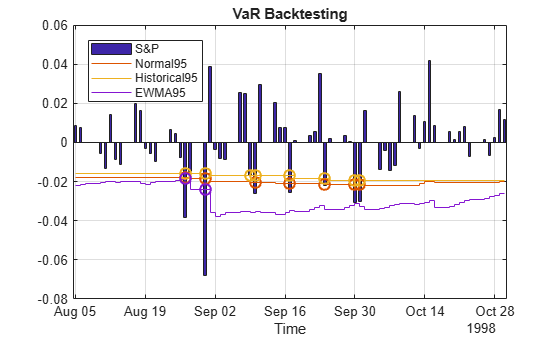A VaR failure or violation happens when the returns have a negative VaR. A closer look around August 27 to August 31 shows a significant dip in the returns. On the dates starting from August 27 onward, the EWMA follows the trend of the returns closely and more accurately. Consequently, EWMA has fewer VaR violations (two (2) violations, yellow diamonds) compared to the Normal Distribution approach (seven (7) violations, blue stars) or the Historical Simulation method (eight (8) violations, red squares).

Besides visual tools, you can use statistical tests for VaR backtesting. In Risk Management Toolbox™, a varbacktest object supports multiple statistical tests for VaR backtesting analysis. In this example, start by comparing the different test results for the normal distribution approach at the 95% and 99% VaR levels.

vbt = varbacktest(ReturnsTest,[Normal95 Normal99],'PortfolioID','S&P','VaRID',... {'Normal95','Normal99'},'VaRLevel',[0.95 0.99]); summary(vbt) 
ans = 2x10 table PortfolioID VaRID VaRLevel ObservedLevel Observations Failures Expected Ratio FirstFailure Missing ___________ __________ ________ _____________ ____________ ________ ________ ______ ____________ _______ "S&P" "Normal95" 0.95 0.94863 1966 101 98.3 1.0275 7 0 "S&P" "Normal99" 0.99 0.98372 1966 32 19.66 1.6277 7 0 

The summary report shows that the observed level is close enough to the defined VaR level. The 95% and 99% VaR levels have at most (1-VaR_level) x N expected failures, where N is the number of observations. The failure ratio shows that the Normal95 VaR level is within range, whereas the Normal99 VaR Level is imprecise and under-forecasts the risk. To run all tests supported in varbacktest, use runtests.

runtests(vbt) 
ans = 2x11 table PortfolioID VaRID VaRLevel TL Bin POF TUFF CC CCI TBF TBFI ___________ __________ ________ ______ ______ ______ ______ ______ ______ ______ ______ "S&P" "Normal95" 0.95 green accept accept accept accept reject reject reject "S&P" "Normal99" 0.99 yellow reject reject accept reject accept reject reject 

The 95% VaR passes the frequency tests, such as traffic light, binomial and proportion of failures tests (tl, bin, and pof columns). The 99% VaR does not pass these same tests, as indicated by the yellow and reject results. Both confidence levels got rejected in the conditional coverage independence, and time between failures independence (cci and tbfi columns). This result suggests that the VaR violations are not independent, and there are probably periods with multiple failures in a short span. Also, one failure may make it more likely that other failures will follow in subsequent days. For more information on the tests methodologies and the interpretation of results, see varbacktest and the individual tests.

Using a varbacktest object, run the same tests on the portfolio for the three approaches at both VaR confidence levels.

vbt = varbacktest(ReturnsTest,[Normal95 Historical95 EWMA95 Normal99 Historical99 ... EWMA99],'PortfolioID','S&P','VaRID',{'Normal95','Historical95','EWMA95',... 'Normal99','Historical99','EWMA99'},'VaRLevel',[0.95 0.95 0.95 0.99 0.99 0.99]); runtests(vbt) 
ans = 6x11 table PortfolioID VaRID VaRLevel TL Bin POF TUFF CC CCI TBF TBFI ___________ ______________ ________ ______ ______ ______ ______ ______ ______ ______ ______ "S&P" "Normal95" 0.95 green accept accept accept accept reject reject reject "S&P" "Historical95" 0.95 yellow accept accept accept accept accept reject reject "S&P" "EWMA95" 0.95 green accept accept accept accept accept reject reject "S&P" "Normal99" 0.99 yellow reject reject accept reject accept reject reject "S&P" "Historical99" 0.99 yellow reject reject accept reject accept reject reject "S&P" "EWMA99" 0.99 red reject reject accept reject accept reject reject 

The results are similar to the previous results, and at the 95% level, the frequency results are generally acceptable. However, the frequency results at the 99% level are generally rejections. Regarding independence, most tests pass the conditional coverage independence test (cci), which tests for independence on consecutive days. Notice that all tests fail the time between failures independence test (tbfi), which takes into account the times between all failures. This result suggests that all methods have issues with the independence assumption.

To better understand how these results change given market conditions, look at the years 2000 and 2002 for the 95% VaR confidence level.

Ind2000 = (year(DatesTest) == 2000); vbt2000 = varbacktest(ReturnsTest(Ind2000),[Normal95(Ind2000) Historical95(Ind2000) EWMA95(Ind2000)],... 'PortfolioID','S&P, 2000','VaRID',{'Normal','Historical','EWMA'}); runtests(vbt2000) 
ans = 3x11 table PortfolioID VaRID VaRLevel TL Bin POF TUFF CC CCI TBF TBFI ___________ ____________ ________ _____ ______ ______ ______ ______ ______ ______ ______ "S&P, 2000" "Normal" 0.95 green accept accept accept accept accept accept accept "S&P, 2000" "Historical" 0.95 green accept accept accept accept accept accept accept "S&P, 2000" "EWMA" 0.95 green accept accept accept accept accept accept accept 
Ind2002 = (year(DatesTest) == 2002); vbt2002 = varbacktest(ReturnsTest(Ind2002),[Normal95(Ind2002) Historical95(Ind2002) EWMA95(Ind2002)],... 'PortfolioID','S&P, 2002','VaRID',{'Normal','Historical','EWMA'}); runtests(vbt2002) 
ans = 3x11 table PortfolioID VaRID VaRLevel TL Bin POF TUFF CC CCI TBF TBFI ___________ ____________ ________ ______ ______ ______ ______ ______ ______ ______ ______ "S&P, 2002" "Normal" 0.95 yellow reject reject accept reject reject reject reject "S&P, 2002" "Historical" 0.95 yellow reject accept accept reject reject reject reject "S&P, 2002" "EWMA" 0.95 green accept accept accept accept reject reject reject 

For the year 2000, all three methods pass all the tests. However, for the year 2002, the test results are mostly rejections for all methods. The EWMA method seems to perform better in 2002, yet all methods fail the independence tests.

To get more insight into the independence tests, look into the conditional coverage independence (cci) and the time between failures independence (tbfi) test details for the year 2002. To access the test details for all tests, run the individual test functions.

cci(vbt2002) 
ans = 3x13 table PortfolioID VaRID VaRLevel CCI LRatioCCI PValueCCI Observations Failures N00 N10 N01 N11 TestLevel ___________ ____________ ________ ______ _________ _________ ____________ ________ ___ ___ ___ ___ _________ "S&P, 2002" "Normal" 0.95 reject 12.591 0.0003877 261 21 225 14 14 7 0.95 "S&P, 2002" "Historical" 0.95 reject 6.3051 0.012039 261 20 225 15 15 5 0.95 "S&P, 2002" "EWMA" 0.95 reject 4.6253 0.031504 261 14 235 11 11 3 0.95 

In the CCI test, the probability p 01 of having a failure at time t, knowing that there was no failure at time t-1 is given byThe probability p 11 of having a failure at time t, knowing that there was failure at time t-1 is given byFrom the N00, N10, N01, N11 columns in the test results, the value of p 01 is at around 5% for the three methods, yet the values of p 11 are above 20%. Because there is evidence that a failure is followed by another failure much more frequently than 5% of the time, this CCI test fails.

In the time between failures independence test, look at the minimum, maximum, and quartiles of the distribution of times between failures, in the columns TBFMin, TBFQ1, TBFQ2, TBFQ3, TBFMax.

tbfi(vbt2002) 
ans = 3x14 table PortfolioID VaRID VaRLevel TBFI LRatioTBFI PValueTBFI Observations Failures TBFMin TBFQ1 TBFQ2 TBFQ3 TBFMax TestLevel ___________ ____________ ________ ______ __________ __________ ____________ ________ ______ _____ _____ _____ ______ _________ "S&P, 2002" "Normal" 0.95 reject 53.936 0.00010087 261 21 1 1 5 17 48 0.95 "S&P, 2002" "Historical" 0.95 reject 45.274 0.0010127 261 20 1 1.5 5.5 17 48 0.95 "S&P, 2002" "EWMA" 0.95 reject 25.756 0.027796 261 14 1 4 7.5 20 48 0.95 

For a VaR level of 95%, you expect an average time between failures of 20 days, or one failure every 20 days. However, the median of the time between failures for the year 2002 ranges between 5 and 7.5 for the three methods. This result suggests that half of the time, two consecutive failures occur within 5 to 7 days, much more frequently than the 20 expected days. Consequently, more test failures occur. For the normal method, the first quartile is 1, meaning that 25% of the failures occur on consecutive days.

### References

Nieppola, O. Backtesting Value-at-Risk Models. Helsinki School of Economics. 2009.

Danielsson, J. Financial Risk Forecasting: The Theory and Practice of Forecasting Market Risk, with Implementation in R and MATLAB®. Wiley Finance, 2012.